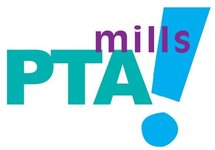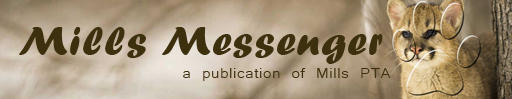## PTA Announcements

 table div table+table+table+table div table{width:100%;padding:0}table div table+table+table+table div table img{width:96.23%;padding:0;float:none}table div table+table+table+table div table td{width:100%;padding:0 1.88% 18px}/* styles */## It’s not too late – you can still join Mills PTA!

 table div table+table+table+table+table+table+table div table{width:100%;padding:0}table div table+table+table+table+table+table+table div table img{width:96.23%;padding:0;float:none}table div table+table+table+table+table+table+table div table td{width:100%;padding:0 1.88% 18px}/* styles */## Birthday Book Club

 /* styles */ Would you like to do something special to honor a birthday or special someone and support the Mills Elementary Library at the same time? You can with the Birthday Book Club! To ‘donate’ a book in honor of your child’s birthday or a special someone, make a \$15 per book donation to Mills Elementary. Your child or favorite staff member will select a book from a collection of new/uncirculated books we have set aside in the library. This will ensure that the book is appropriate for our collection and is published in library binding. A special bookplate will be made after their book is selected, and placed in the front cover of the book. If permission is granted, a photo of your child will be displayed on the Library's Birthday Book Club Bulletin Board. Your child will be the first person to check out the new book! And it doesn’t have to be on their birthday! To participate in this year's Birthday Book Club, please fill out and return this form to the library. Forms can also be found in the pocket at the bottom of the Birthday Book Club bulletin board. Thank you for supporting the Mills Elementary Library! Your librarians, Jan Higgs and Sarah Rasmussen
 table div table+table+table+table+table+table+table+table+table+table div table{width:100%;padding:0}table div table+table+table+table+table+table+table+table+table+table div table img{width:96.23%;padding:0;float:none}table div table+table+table+table+table+table+table+table+table+table div table td{width:100%;padding:0 1.88% 18px}/* styles */## Mills Dirt Club Dates

Embrace the heat! The Dirt Club (aka Campus Landscaping and Beautification) will be meeting up on the following dates. We'll concentrate on some lighter-duty cleanup and maintenance—weeding, trimming, clearing rocks, cleaning up beds, touching up sidewalk paint, etc.

No experience necessary, just a willingness to get dirty! Come for part or all of the time window...all help is appreciated. Meet in the parking lot in front of the school. You probably want to bring some water and a favorite pair of work gloves (we should have extra gloves if needed).

▪ September 12, 6:30PM - Sunset
▪ September 21, 8 - 11AM
 ▪ September 12, 6:30PM - Sunset
 ▪ September 21, 8 - 11AM
 table div table+table+table+table+table+table+table+table+table+table+table+table+table div table{width:100%;padding:0}table div table+table+table+table+table+table+table+table+table+table+table+table+table div table img{width:96.23%;padding:0;float:none}table div table+table+table+table+table+table+table+table+table+table+table+table+table div table td{width:100%;padding:0 1.88% 18px}/* styles */## Library Volunteer Orientation

 table div table+table+table+table+table+table+table+table+table+table+table+table+table+table+table+table div table{width:100%;padding:0}table div table+table+table+table+table+table+table+table+table+table+table+table+table+table+table+table div table img{width:96.23%;padding:0;float:none}table div table+table+table+table+table+table+table+table+table+table+table+table+table+table+table+table div table td{width:100%;padding:0 1.88% 18px}/* styles */## Reflections

 table div table+table+table+table+table+table+table+table+table+table+table+table+table+table+table+table+table+table+table div table{width:100%;padding:0}table div table+table+table+table+table+table+table+table+table+table+table+table+table+table+table+table+table+table+table div table img{width:96.23%;padding:0;float:none}table div table+table+table+table+table+table+table+table+table+table+table+table+table+table+table+table+table+table+table div table td{width:100%;padding:0 1.88% 18px}/* styles */## Math Pentathlon

 table div table+table+table+table+table+table+table+table+table+table+table+table+table+table+table+table+table+table+table+table+table+table div table{width:100%;padding:0}table div table+table+table+table+table+table+table+table+table+table+table+table+table+table+table+table+table+table+table+table+table+table div table img{width:96.23%;padding:0;float:none}table div table+table+table+table+table+table+table+table+table+table+table+table+table+table+table+table+table+table+table+table+table+table div table td{width:100%;padding:0 1.88% 18px}/* styles */## Mills 1st Spirit Night at Torchy's Tacos - Escarpment

 table div table+table+table+table+table+table+table+table+table+table+table+table+table+table+table+table+table+table+table+table+table+table+table+table div table,table.module-23{width:61.32%;float:right;padding:0}table div table+table+table+table+table+table+table+table+table+table+table+table+table+table+table+table+table+table+table+table+table+table+table+table div table a{border:0 none;text-decoration:none}table div table+table+table+table+table+table+table+table+table+table+table+table+table+table+table+table+table+table+table+table+table+table+table+table div table img{width:100%!important;border:0 none;text-decoration:none}table div table+table+table+table+table+table+table+table+table+table+table+table+table+table+table+table+table+table+table+table+table+table+table+table div table td{width:100%;padding:0 0 20px 20px}/* styles */ It's TACO TUESDAY Y'all!!! Come on out and support your school and PTA by getting some grub at Torchy's off Escarpment from 4pm-8pm. Make sure to either bring a flyer, or tell the cashier that you are with Mills Elementary so that Mills PTA will get a percentage of the proceeds. The money goes back into the school for use where it is needed!! It is a win win for everyone, so make sure to mark it on your calendar!! TACO TUESDAY at TORCHY'S (escarpment location only) 9/17/2019 from 4pm-8pm!!!
 table div table+table+table+table+table+table+table+table+table+table+table+table+table+table+table+table+table+table+table+table+table+table+table+table+table div table{width:100%;padding:0}table div table+table+table+table+table+table+table+table+table+table+table+table+table+table+table+table+table+table+table+table+table+table+table+table+table div table img{width:96.23%;padding:0;float:none}table div table+table+table+table+table+table+table+table+table+table+table+table+table+table+table+table+table+table+table+table+table+table+table+table+table div table td{width:100%;padding:0 1.88% 18px}/* styles */## Online Directory: Change of Plans!

 /* styles */ We are not going to have an online directory this year. With the popularity of email, text messaging, and social media platforms like Facebook, usage of our online directory had been steadily declining in recent years; in addition, the tool we used (MySchoolAnywhere) was acquired by another company this year, which meant all of our users would need to register for and learn to use a new tool. So, the PTA board decided this is the right time to end our online directory. As alternatives, we would encourage you to use the Facebook group for families and staff (https://www.facebook.com/groups/28601035708/), form or join a group for your student's classroom, and contact your student's teacher and room mom or dad to help coordinate communication among parents. If you have any questions or need tips or ideas on how to connect with other Mills families, please email directory@millspta.org. Thanks!
 table div table+table+table+table+table+table+table+table+table+table+table+table+table+table+table+table+table+table+table+table+table+table+table+table+table+table+table+table div table{width:100%;padding:0}table div table+table+table+table+table+table+table+table+table+table+table+table+table+table+table+table+table+table+table+table+table+table+table+table+table+table+table+table div table img{width:96.23%;padding:0;float:none}table div table+table+table+table+table+table+table+table+table+table+table+table+table+table+table+table+table+table+table+table+table+table+table+table+table+table+table+table div table td{width:100%;padding:0 1.88% 18px}/* styles */## Be Kind 21 Challenge

Mills is participating in the Be Kind 21 Challenge for the first 21 days of September! Encourage your child to participate beginning on Sunday, September 1st, to Saturday, September 21st. You can participate, too. It's important to be kind and to spread kindness. See the 21 daily challenges listed here!

 table div table+table+table+table+table+table+table+table+table+table+table+table+table+table+table+table+table+table+table+table+table+table+table+table+table+table+table+table+table+table+table div table{width:100%;padding:0}table div table+table+table+table+table+table+table+table+table+table+table+table+table+table+table+table+table+table+table+table+table+table+table+table+table+table+table+table+table+table+table div table img{width:96.23%;padding:0;float:none}table div table+table+table+table+table+table+table+table+table+table+table+table+table+table+table+table+table+table+table+table+table+table+table+table+table+table+table+table+table+table+table div table td{width:100%;padding:0 1.88% 18px}/* styles */## Affiliate News

 table div table+table+table+table+table+table+table+table+table+table+table+table+table+table+table+table+table+table+table+table+table+table+table+table+table+table+table+table+table+table+table+table+table div table{width:100%;padding:0}table div table+table+table+table+table+table+table+table+table+table+table+table+table+table+table+table+table+table+table+table+table+table+table+table+table+table+table+table+table+table+table+table+table div table img{width:96.23%;padding:0;float:none}table div table+table+table+table+table+table+table+table+table+table+table+table+table+table+table+table+table+table+table+table+table+table+table+table+table+table+table+table+table+table+table+table+table div table td{width:100%;padding:0 1.88% 18px}/* styles */Back-to-school is in full swing at Young Rembrandts, as we present drawing lessons that encompass a fascinating world of color, pattern, and design. All-new lessons including a backpack still-life composition, detailed gumball machine, decorative Egyptian headdress and seasonally inspired drawings will strengthen students’ drawing, coloring and classroom learning skills. All this and more await your child in a Young Rembrandts class.

https://www.amilia.com/store/en/yr-westaustin/api/Activity/Detail?activityId=k4JjgVy

 table div table+table+table+table+table+table+table+table+table+table+table+table+table+table+table+table+table+table+table+table+table+table+table+table+table+table+table+table+table+table+table+table+table+table+table div table{width:100%;padding:0}table div table+table+table+table+table+table+table+table+table+table+table+table+table+table+table+table+table+table+table+table+table+table+table+table+table+table+table+table+table+table+table+table+table+table+table div table img{width:96.23%;padding:0;float:none}table div table+table+table+table+table+table+table+table+table+table+table+table+table+table+table+table+table+table+table+table+table+table+table+table+table+table+table+table+table+table+table+table+table+table+table div table td{width:100%;padding:0 1.88% 18px}/* styles */Mills has a great opportunity coming after school! Is your child always coming up with ideas? Does your child want to start their own business one day? Then, this may be the perfect after-school activity. Start-Up Kids Club is an innovative, hands-on youth entrepreneurship program. Students K through 5th are inspired to bring their ideas to life with support from local community experts and "grown-up" entrepreneurs.

With a two-market approach, students are given the opportunity to adapt, iterate, and pivot based on outcomes from one market to the next. Start-Up Kids Club teachers using both qualitative and quantitative assessment to encourage reflection and perception of both success and failure as valuable information. Start-Up Kids started here at Mills last semester, and they're back again.

Check out the link at https://startupkidsclub.com/austin/ to register today. It's not too late! Classes begin next week on Tuesday, September 3rd.

 table div table+table+table+table+table+table+table+table+table+table+table+table+table+table+table+table+table+table+table+table+table+table+table+table+table+table+table+table+table+table+table+table+table+table+table+table+table div table{width:100%;padding:0}table div table+table+table+table+table+table+table+table+table+table+table+table+table+table+table+table+table+table+table+table+table+table+table+table+table+table+table+table+table+table+table+table+table+table+table+table+table div table img{width:96.23%;padding:0;float:none}table div table+table+table+table+table+table+table+table+table+table+table+table+table+table+table+table+table+table+table+table+table+table+table+table+table+table+table+table+table+table+table+table+table+table+table+table+table div table td{width:100%;padding:0 1.88% 18px}/* styles */table div table+table+table+table+table+table+table+table+table+table+table+table+table+table+table+table+table+table+table+table+table+table+table+table+table+table+table+table+table+table+table+table+table+table+table+table+table+table+table div table{width:100%;padding:0}table div table+table+table+table+table+table+table+table+table+table+table+table+table+table+table+table+table+table+table+table+table+table+table+table+table+table+table+table+table+table+table+table+table+table+table+table+table+table+table div table img{width:96.23%;padding:0;float:none}table div table+table+table+table+table+table+table+table+table+table+table+table+table+table+table+table+table+table+table+table+table+table+table+table+table+table+table+table+table+table+table+table+table+table+table+table+table+table+table div table td{width:100%;padding:0 1.88% 18px}/* styles */## Need to get your message out?

 /* styles */ Submit info to the Mills Messenger. Want to see the Mills Calendar on your Google calendar? Add Mills Calendar to my Google Calendar. Have a friend who isn't getting the Messenger but wants to? Send them this link to sign up: https://madmimi.com/signups/join/7059
 table div table+table+table+table+table+table+table+table+table+table+table+table+table+table+table+table+table+table+table+table+table+table+table+table+table+table+table+table+table+table+table+table+table+table+table+table+table+table+table+table+table+table div table{width:100%;padding:0}table div table+table+table+table+table+table+table+table+table+table+table+table+table+table+table+table+table+table+table+table+table+table+table+table+table+table+table+table+table+table+table+table+table+table+table+table+table+table+table+table+table+table div table img{width:96.23%;padding:0;float:none}table div table+table+table+table+table+table+table+table+table+table+table+table+table+table+table+table+table+table+table+table+table+table+table+table+table+table+table+table+table+table+table+table+table+table+table+table+table+table+table+table+table+table div table td{width:100%;padding:0 1.88% 18px}/* styles */